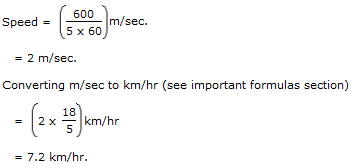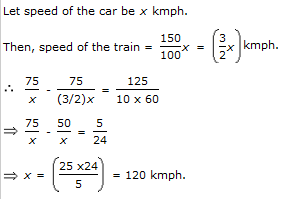1.

A person crosses a 600 m long street in 5 minutes. What is his speed in km per hour?

 A. 3.6 B. 7.2 C. 8.4 D. 10
Explanation:

2.

A person crosses a 600 m long street in 5 minutes. What is his speed in km per hour?

 A. 3.6 B. 7.2 C. 8.4 D. 10
Explanation:3.

An aeroplane covers a certain distance at a speed of 240 kmph in 5 hours. To cover the same distance in 1 2/3 hours, it must travel at a speed of:

 A. 300 kmph B. 360 kmph C. 600 kmph D. 720 kmph
Explanation: Distance = (240 x 5) = 1200 km.

Speed = Distance/Time

Speed = 1200/(5/3) km/hr. [We can write 1 2/3 hours as 5/3 hours]

Required speed = 1200 x 3/5 km/hr = 720 km/hr.

4.

If a person walks at 14 km/hr instead of 10 km/hr, he would have walked 20 km more. The actual distance travelled by him is:

 A. 50 km B. 56 km C. 70 km D. 80 km
Explanation: Let the actual distance travelled be x km.

Then, x/10 = (x + 20)/14

14x = 10x + 200

4x = 200

x = 50 km

5.

A train can travel 50% faster than a car. Both start from point A at the same time and reach point B 75 kms away from A at the same time. On the way, however, the train lost about 12.5 minutes while stopping at the stations. The speed of the car is:

 A. 100 kmph B. 110 kmph C. 120 kmph D. 130 kmph
Explanation: